# Introduction to gravity and magnetic field classes¶

pyshtools has dedicated classes for working with gravitational and magnetic fields. These classes extend the functionality of the classes SHCoeffs and SHGrid by adding several standard operations related to potential fields. Specific operations include the computation of the geoid, the computation of the three vector components of the gravitational and magnetic fields, the computation of the gravity and magnetic field tensor and eigenvalues, and the computation of the gravitational potential from relief along an interface.

To start using the gravity and magnetic field classes, first import the standard matplotlib package, the pyshtools package, and the pyshtools subpackage constant:

In :
from __future__ import print_function # only necessary if using Python 2.x
%matplotlib inline

import matplotlib.pyplot as plt
import pyshtools
from pyshtools import constant

In :
pyshtools.utils.figstyle(rel_width=0.75)
%config InlineBackend.figure_format = 'retina'  # if you are not using a retina display, comment this line


## Gravity field classes¶

Operations on gravity fields are performed using the classes SHGravCoeffs, SHGravGrid, SHGravTensor, and SHGeoid. In gereral, the coefficients are stored in an SHGravCoeffs class instance, and this instance is used to initialize the other classes with global grids.

There are several ways to initialize an SHGravCoeffs class instance:

• SHGravCoeffs.from_zeros() - Set the degree 0 term to 1 and all other coefficients to zero.
• SHGravCoeffs.from_array() - Import a numpy array of spherical harmonic coefficients.
• SHGravCoeffs.from_file() - Read coefficients from a file.
• SHGravCoeffs.from_random() - Initialize the coefficients as random variables with a given power spectrum.
• SHGravCoeffs.from_shape() - Compute the gravitational potential coefficients from relief along an interface.

Unlike SHCoeffs, when initializing an SHGravCoeffs class instance, it is necessary to specify the attributes gm, r0, and optionally omega, for the GM, reference radius of the coefficients, and the angular rotation rate of the body.

We start by reading the gravitational potential coefficients and errors for the planet Mars. We make use of the model GMM-3 of Genova et al. (2016), where r0 and gm are set by the first two elements of the file's header line. If the file didn't contain a header with this information, these parameters could be specified explicitly by using the keywords gm and r0. We note that the header line of this file uses units of kilometers instead of meters, so we convert the values in the header to meters by specifiying header_units='km':

In :
clm = pyshtools.SHGravCoeffs.from_file('../ExampleDataFiles/gmm3_120_sha.tab', errors=True, header_units='km')


Immediately, we receive a warning message because the file did not set the degree-0 spherical harmonic coefficient: when this occurs, pyshtools will automatically sets this value to unity. We can inspect this class instance by using the info() method, by print(clm), or simply by entering the name of the class instance at the prompt:

In :
clm.info()

kind = 'real'
normalization = '4pi'
csphase = 1
lmax = 120
GM (m3 / s2) = 42828372854187.75
r0 (m) = 3396000.0
Omega (rad / s) = None
errors are set: True
header = ['3.3960000000000000E+03', '0.4282837285418775E+05', '0.2380000000000000E+04', '120', '120', '1', '0.0000000000000000E+00', '0.0000000000000000E+00']


Using the constant submodule, we set the angular rotation rate of the planet, which is necessary when calculating the normal gravity and geoid. We also define variables for the planet's reference ellipsoid, such as the semimajor axis a, the flattening f, and the reference potential u0:

In :
clm.set_omega(constant.omega_mars.value)

a = constant.a_mars.value
f = constant.f_mars.value
u0 = constant.u0_mars.value


The spectrum of the function can be plotted using the methods plot_spectrum() and plot_spectrum2d(). Unlike SHCoeffs class instances, we have the option of plotting the power spectrum of the geoid (default), potential, radial gravity, or total gravitational field as specified by the optional parameter function. The total spectrum is analogous to the Lowes-Mauersberger spectrum of magnetic fields.

In :
fig1, ax1 = clm.plot_spectrum(function='geoid', show=False)
fig2, ax2 = clm.plot_spectrum2d(function='total', show=False)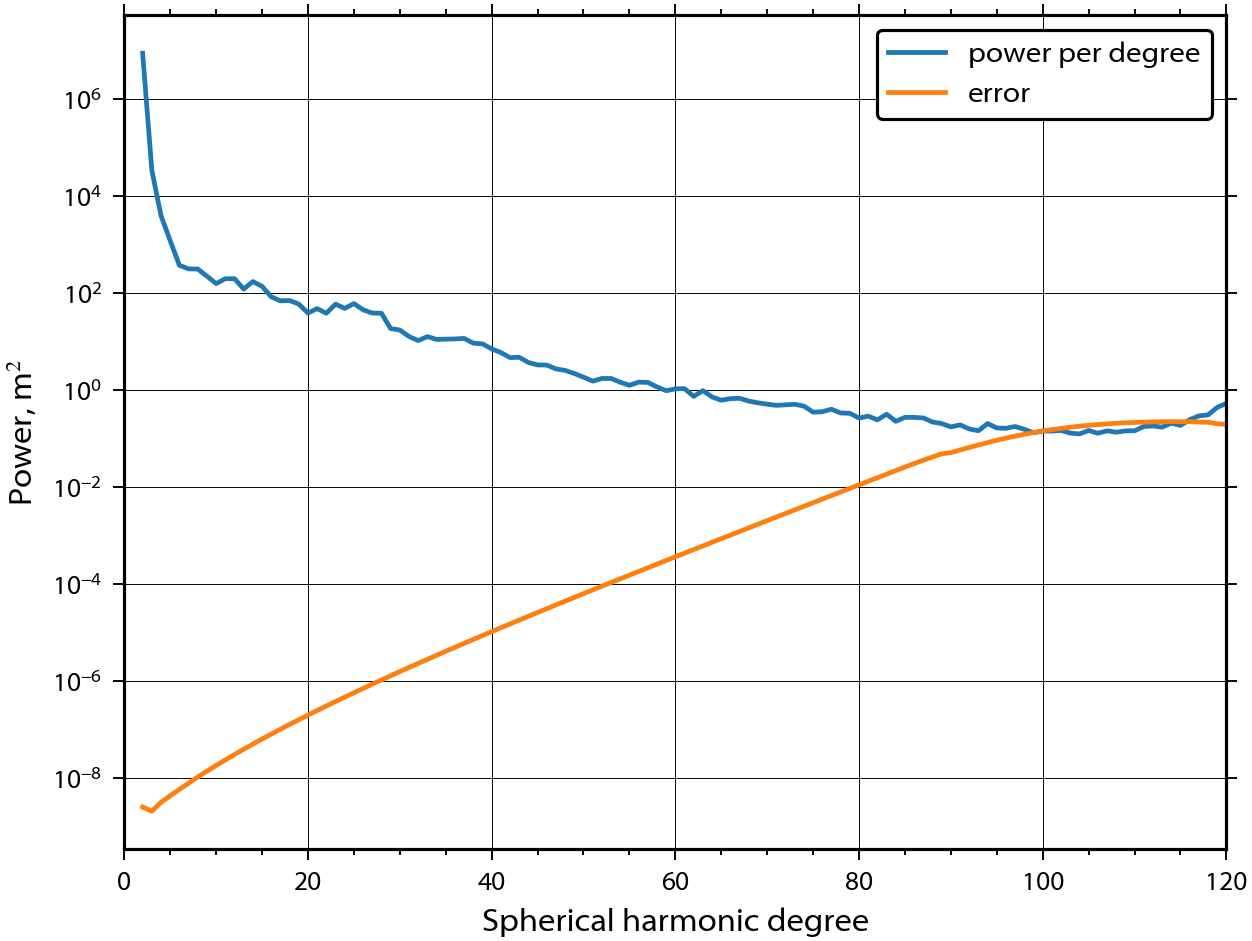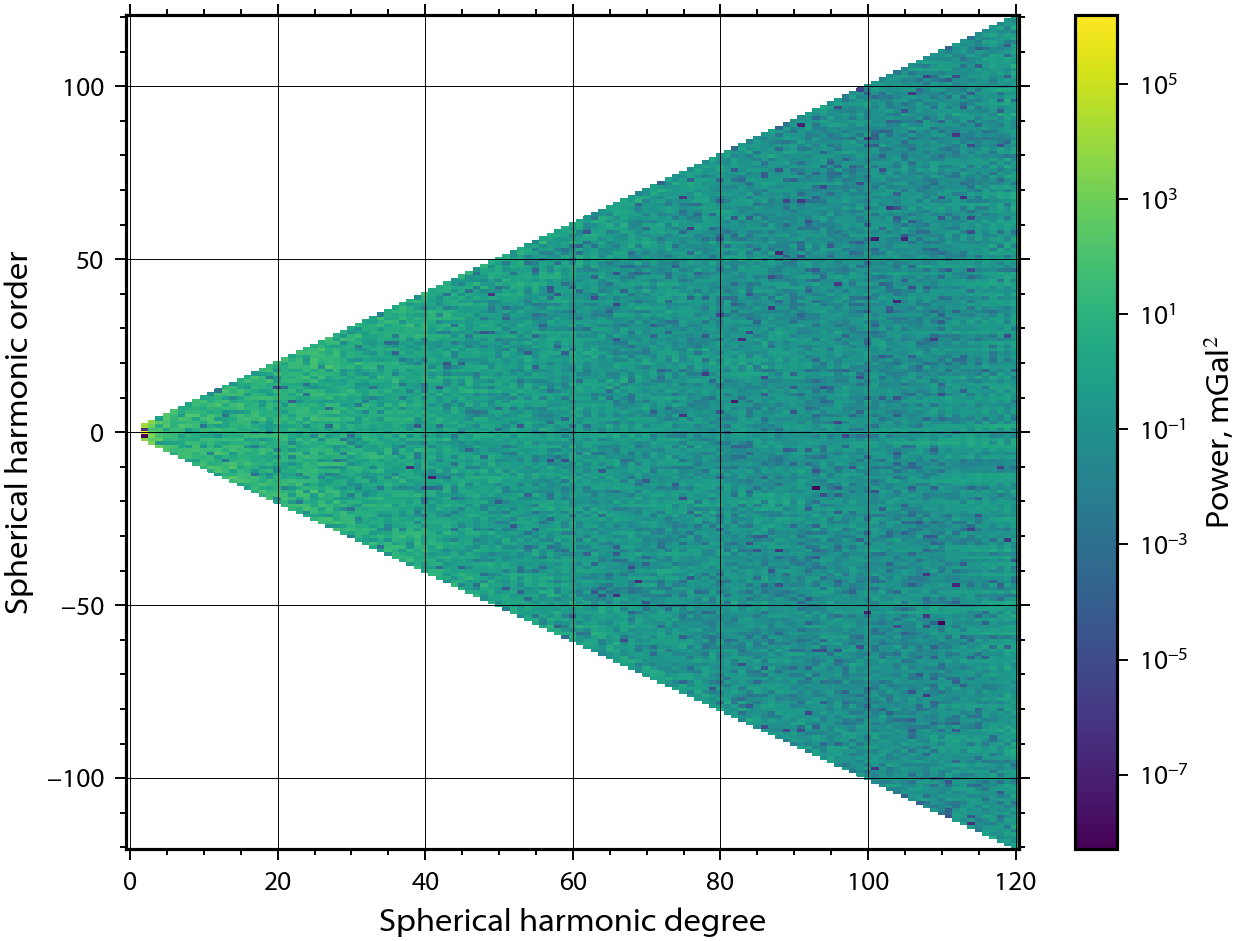If the errors of the gravitational potential are set, plot_spectrum() will compute automatically and plot the error spectrum. For plot_spectrum2d(), the errors can be plotted by specifying errors=True. By default, the power associated with the degree 0 and 1 terms will not plotted, because the degree 0 term is orders of magnitude larger than the others, and the degree-1 terms are zero when in center-of-mass coordinates.

Next, we use the method expand() to calculate grids of the gravitational potential, the three vector components of the gravity field, and the total gravity disturbance. By default, these values are calculated on a sphere whose radius is equal to the reference radius r0. By specifying the parameters a and f, these quantities will instead be calculated on a flattened ellipsoid. In this case, it should be emphasized that the vector components correspond to the spherical coordinate unit vectors $\hat{r}$, $\hat{\theta}$, and $\hat{\phi}$, and not components that are perpendicular to the reference ellipsoid. By default, the normal gravity is removed from the total gravitational acceleration, yielding the gravity disturbance.

The expand() method returns an SHGravGrid class instance. This class stores each of the five grids, rad, theta, phi, total, and pot as SHGrid class instances. The four gravity grids can be plotted together using the method plot():

In :
grav = clm.expand(lmax=95, a=a, f=f)
fig2, ax2 = grav.plot(show=False)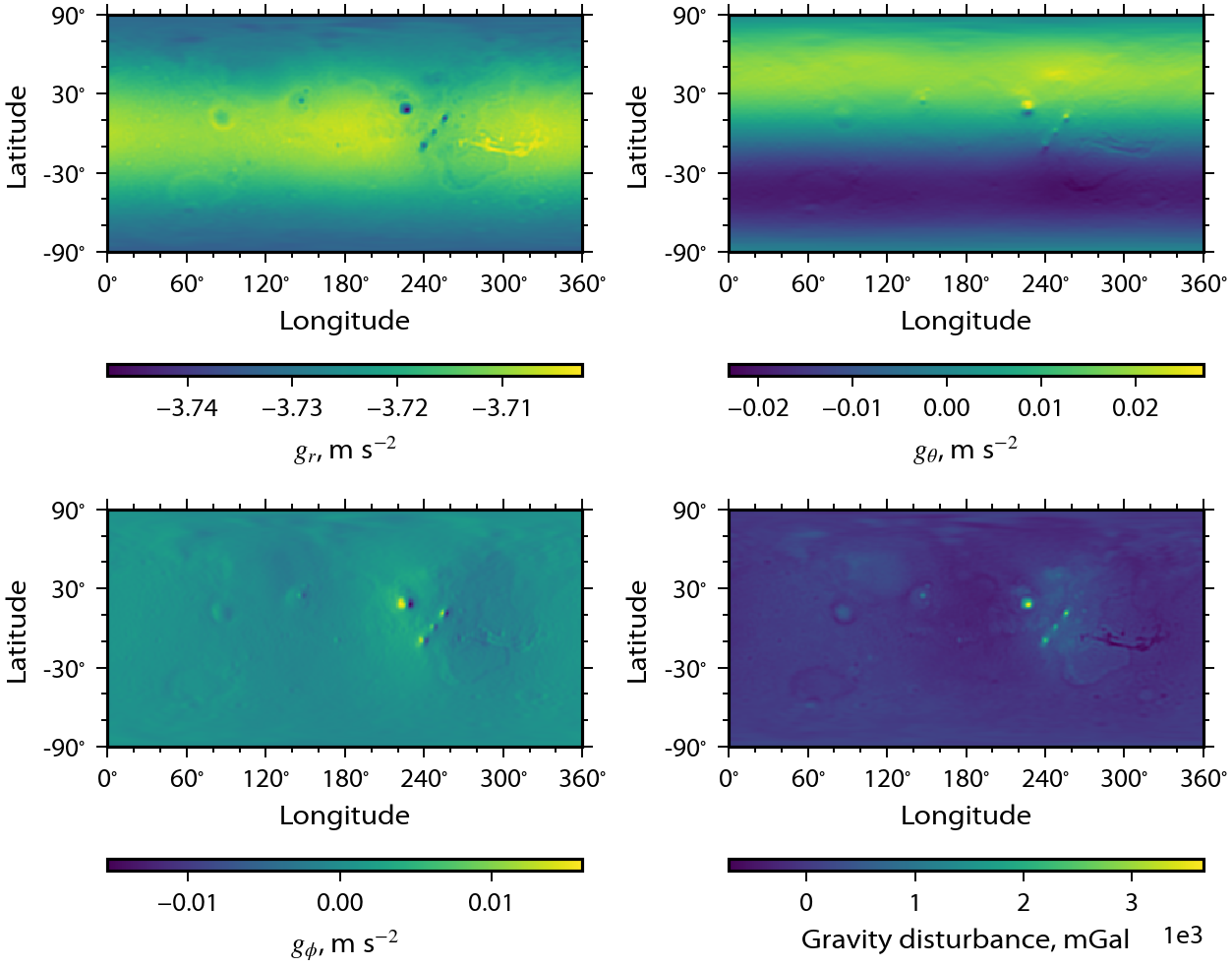Individual maps can be plotted using the corresponding methods plot_rad(), plot_theta(), plot_phi(), plot_total(), and plot_pot(). Here, we plot the total gravity disturbance, and use the parameters vmin, vmax, and cmap to set the minimum and maximum values and color scale, respectively:

In :
fig3, ax3 = grav.plot_total(vmin=-400, vmax=600, cmap='RdYlBu_r', show=False)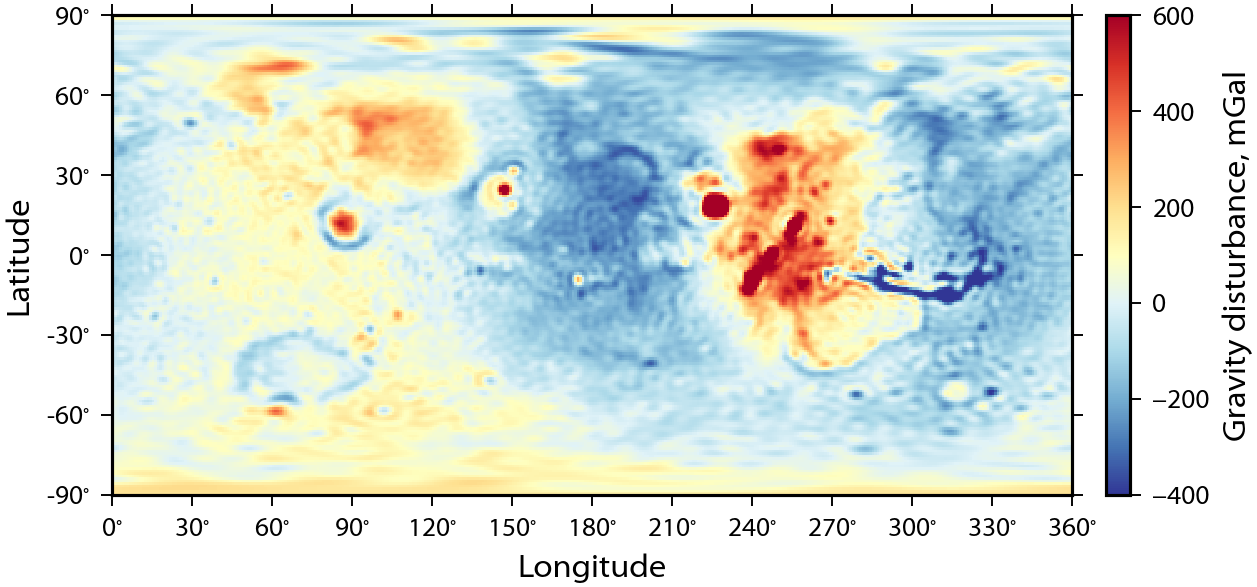Remember, you should never use the 'rainbow' and 'jet' colormaps!

The geoid is computed using the geoid() method. By specifying the reference potential (in m$^2$/s$^2$), the height is calculted with respect to a sphere of reference radius r0. This method returns an SHGeoid class instance, where the geoid map is stored internally as an SHGrid class instance. Here, we compute the geoid on a grid that has a finer resolution than the potential coefficients by specifying the optional variable lmax. The reason for doing so is to compare this map directly to a higher resolution map of the shape of the planet later:

In :
mars_geoid = clm.geoid(u0, lmax=719)
fig4, ax4 = mars_geoid.plot(cmap='RdYlBu_r', show=False)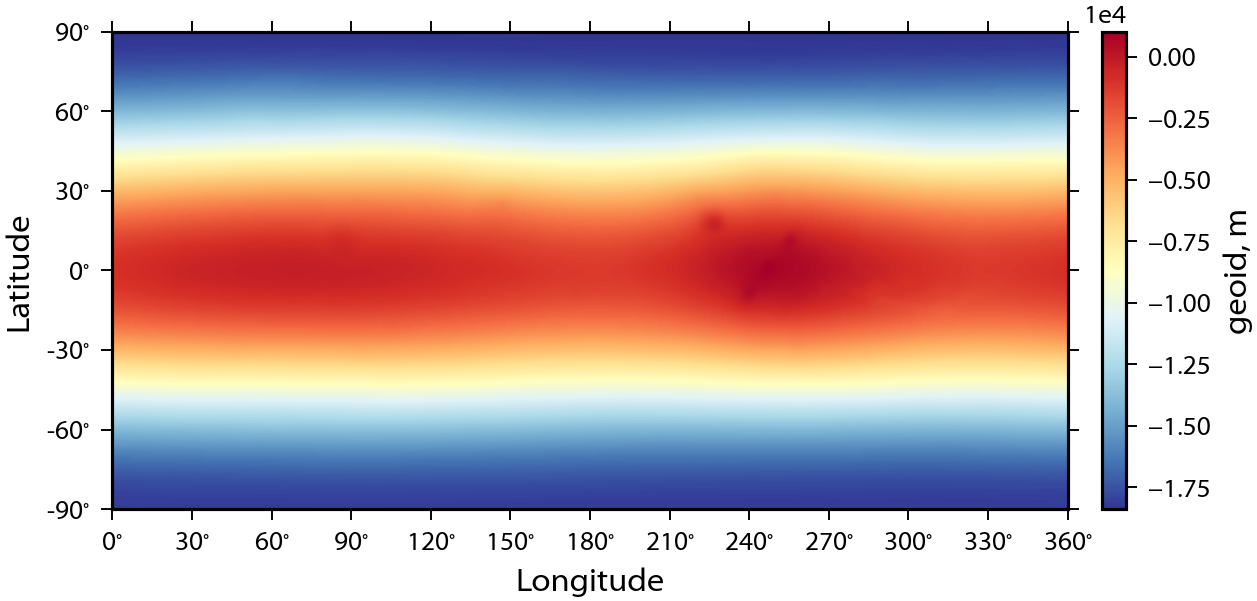In this image, the geoid height largely reflects the rotational flattening of the planet. To calculate the geoid height with respect to a flattened ellipsoid, it is only necessary to specify the optional parameters a and f:

In :
mars_geoid_ellipsoid = clm.geoid(u0, a=a, f=f, lmax=719)
fig5, ax5 = mars_geoid_ellipsoid.plot(vmin=-750, vmax=1200, cmap='RdYlBu_r', show=False)